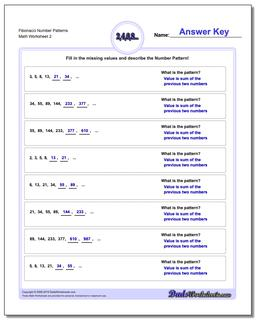# Math Worksheets: Number Patterns: Number Patterns: Fibonacci Number Patterns (Second Worksheet)## Fibonacci Number Patterns (Second Worksheet)

PropertyValue
DescriptionFibonacci Number Patterns: Fibonacci and Fibonacci-like number pattern problems, where the next values in a sequence are dependent on prior values. (Second Worksheet)
Resource TypeWorksheet